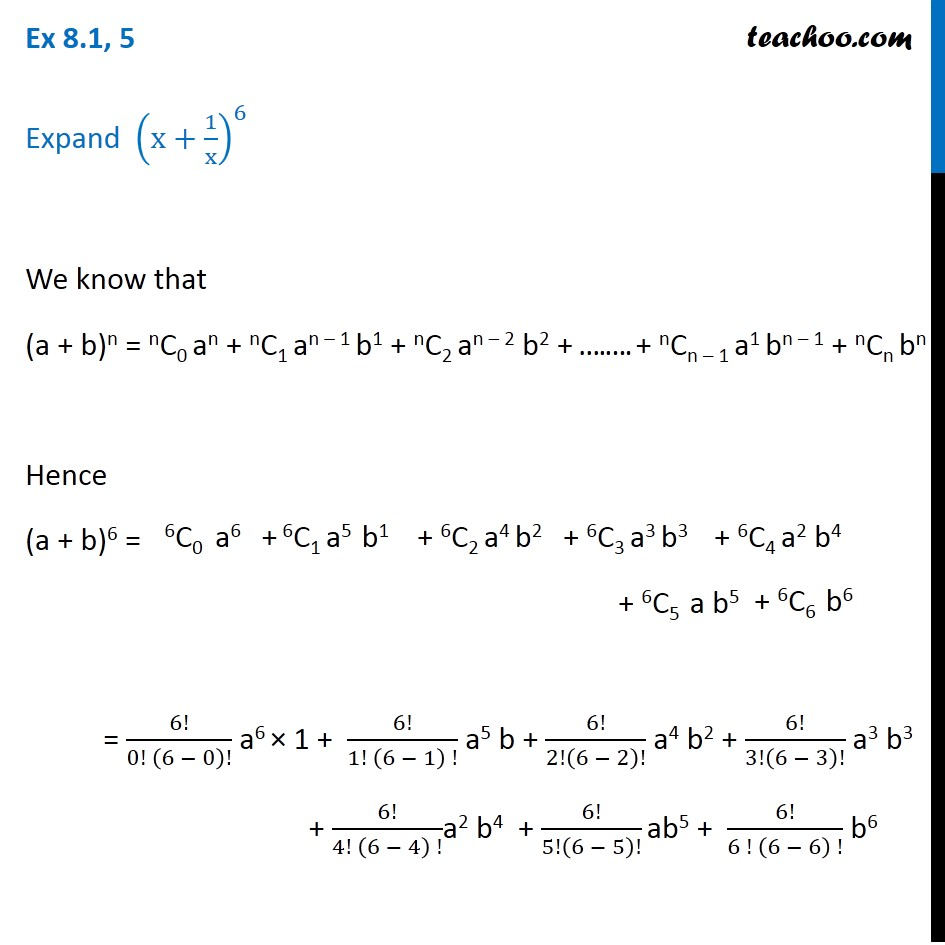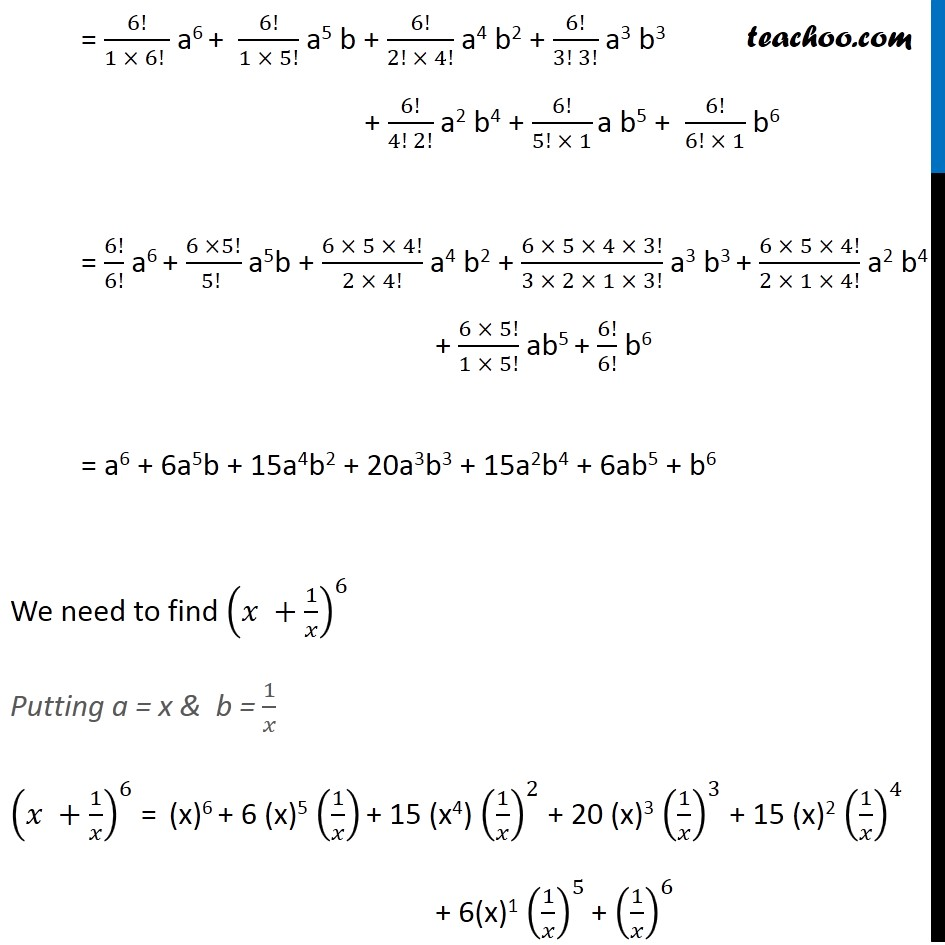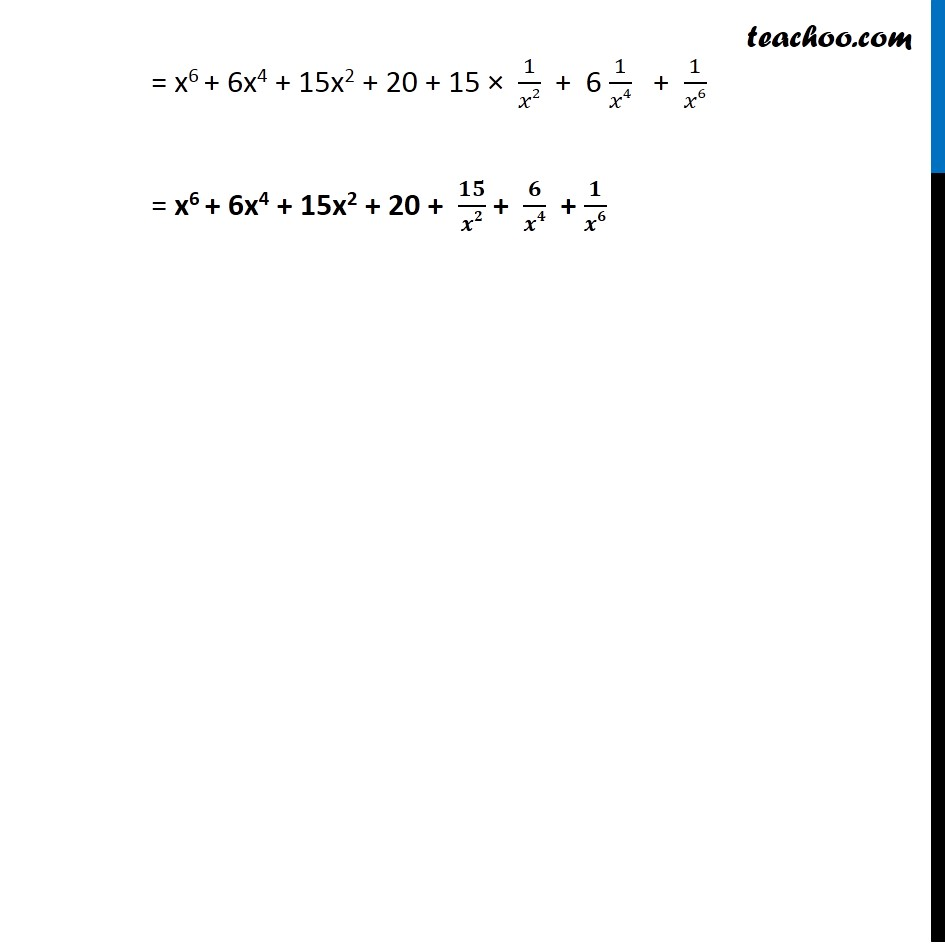Ex 7.1

Chapter 7 Class 11 Binomial Theorem
Serial order wiseLearn in your speed, with individual attention - Teachoo Maths 1-on-1 Class

### Transcript

Ex 7.1, 5 Expand (x+1/x)^6 We know that (a + b)n = nC0 an + nC1 an – 1 b1 + nC2 an – 2 b2 + ….…. + nCn – 1 a1 bn – 1 + nCn bn Hence (a + b)6 = = 6!/(0! (6 − 0)!) a6 × 1 + 6!/(1! (6 − 1) !) a5 b + 6!/2!(6 − 2)! a4 b2 + 6!/3!(6 − 3)! a3 b3 + 6!/(4! (6 − 4) !)a2 b4 + 6!/5!(6 − 5)! ab5 + 6!/(6 ! (6 − 6) !) b6 = 6!/(1 × 6! ) a6 + 6!/(1 × 5!) a5 b + 6!/(2! × 4!) a4 b2 + 6!/(3! 3!) a3 b3 + 6!/(4! 2!) a2 b4 + 6!/(5! × 1) a b5 + 6!/(6! × 1) b6 = 6!/6! a6 + (6 ×5!)/(5! ) a5b + (6 × 5 × 4!)/(2 × 4!) a4 b2 + (6 × 5 × 4 × 3!)/(3 × 2 × 1 × 3!) a3 b3 + (6 × 5 × 4!)/(2 × 1 × 4!) a2 b4 + (6 × 5!)/(1 × 5!) ab5 + 6!/6! b6 = a6 + 6a5b + 15a4b2 + 20a3b3 + 15a2b4 + 6ab5 + b6 We need to find (𝑥 +1/𝑥)^6 Putting a = x & b = 1/𝑥 (𝑥 +1/𝑥)^6 = (x)6 + 6 (x)5 (1/𝑥) + 15 (x4) (1/𝑥)^2 + 20 (x)3 (1/𝑥)^3 + 15 (x)2 (1/𝑥)^4 + 6(x)1 (1/𝑥)^5 + (1/𝑥)^6 = x6 + 6x4 + 15x2 + 20 + 15 × 1/𝑥2 + 6 1/𝑥4 + 1/𝑥6 = x6 + 6x4 + 15x2 + 20 + 𝟏𝟓/𝒙𝟐 + 𝟔/𝒙𝟒 + 𝟏/𝒙𝟔